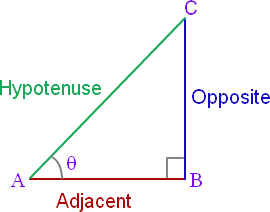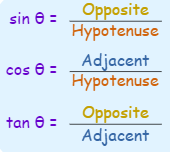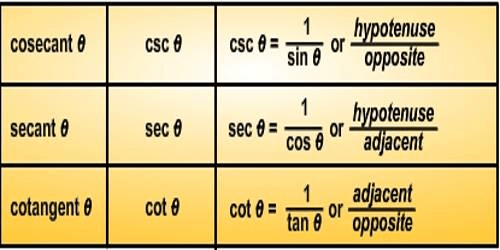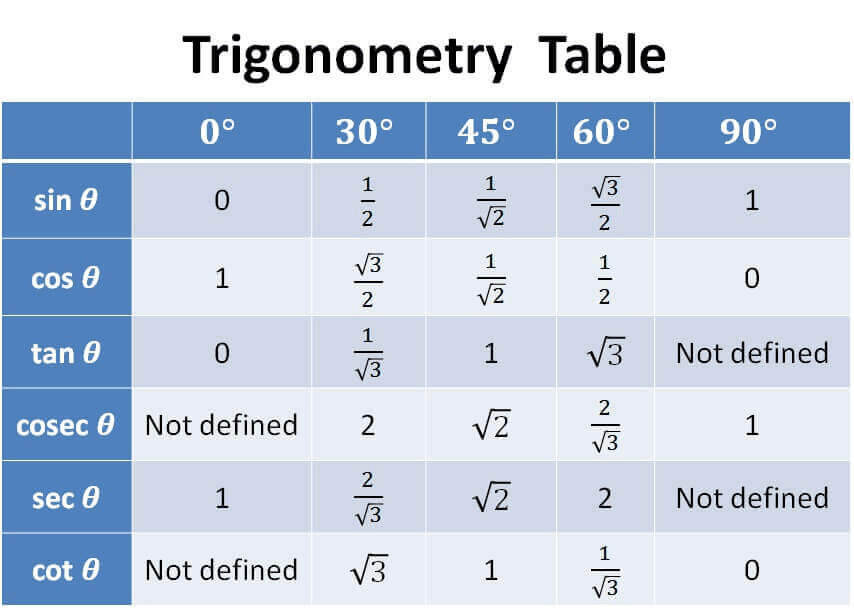Important Definitions & Formulas: Introduction to Trigonometry

# Important Definitions & Formulas: Introduction to Trigonometry - Notes | Study Mathematics (Maths) Class 10 - Class 10

 Table of contentsDefinitions and Formulas1 Crore+ students have signed up on EduRev. Have you?

Definitions and Formulas

• An angle is positive if it is measured in the anticlockwise direction and negative if it is measured in the clockwise direction.
• In a right triangle ABC, right-angled at BReciprocal Relations• If one of the trigonometric ratios of an acute angle is known, the remaining trigonometric ratios can be determined.
• Two angles are said to be complementary if their sum is 90°, and each one of them is called the complement of the other.
sin (90° - θ) = cos θ
cos (90° - θ) = sin θ
tan (90° - θ) = cot θ
cot (90° - θ) = tan θ
sec (90° - θ) = cosec θ
cosec (90° - θ) = sec θ
Trigonometric Identities

An equation with trigonometric ratios of an angle θ, which is true for all values of ‘θ’, for which the given trigonometric ratios are defined, is called an identity.
Three fundamental trigonometric identities are
(a) sin2θ + cos2θ = 1
(b) sec2θ = 1 + tan2θ
(c) cosec2θ = 1 + cot2θ

Table: Values of trigonometric ratios of 0°, 30°, 45°, 60° and 90°The document Important Definitions & Formulas: Introduction to Trigonometry - Notes | Study Mathematics (Maths) Class 10 - Class 10 is a part of the Class 10 Course Mathematics (Maths) Class 10.
All you need of Class 10 at this link: Class 10

## Mathematics (Maths) Class 10

53 videos|403 docs|138 tests
 Use Code STAYHOME200 and get INR 200 additional OFF

## Mathematics (Maths) Class 10

53 videos|403 docs|138 tests

Track your progress, build streaks, highlight & save important lessons and more!

,

,

,

,

,

,

,

,

,

,

,

,

,

,

,

,

,

,

,

,

,

;